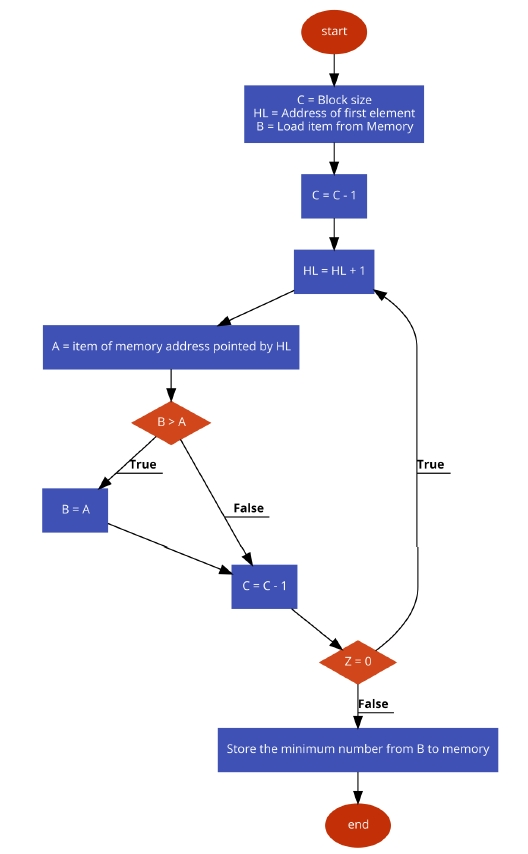# 8085 Program to find the smallest number

In this program we will see how to find the smallest number from a block of bytes using 8085.

### Problem Statement

Write 8085 Assembly language program to find the smallest number from a block of bytes.

### Discussion

In this program the data are stored at location 8001H onwards. The 8000H is containing the size of the block. After executing this program, it will return the smallest number and store it at location 9000H.

Logic is simple, we are taking the first number at register B to start the job. In each iteration we are getting the number from memory and storing it into register A. Then if B > A, then we simply update the value of B with A, otherwise go for the next iteration. Thus we can find the smallest number in a block of bytes.

......
800006
800155
800222
800344
800411
800533
800666
......

### Flow Diagram### Program

F00021, 00, 80
LXI H,8000HPoint to get array size
F0034E
MOV C, MGet the sizeof array
F00423
INX H   Point to actual array
F00546
MOV B, MLoad the first number into B
F0060D
DCR C   Decrease C
F00723LOOPINX H   Point to next location
F0087E
MOV A, MGet the next number from memory to Acc
F009B8
CMP B   Compare Acc and B
JNC SKIPif B <= A,then skip
F00D47
MOV B,AIf CY is 1, update B
F00E0DSKIPDCR C   Decrease C
F00FC2, 07, F0
JNZ LOOP    When count is not 0, go to LOOP
F01221, 00, 90
F01570
MOV M,BStore the minimum number
F01676
HLTTerminate the program